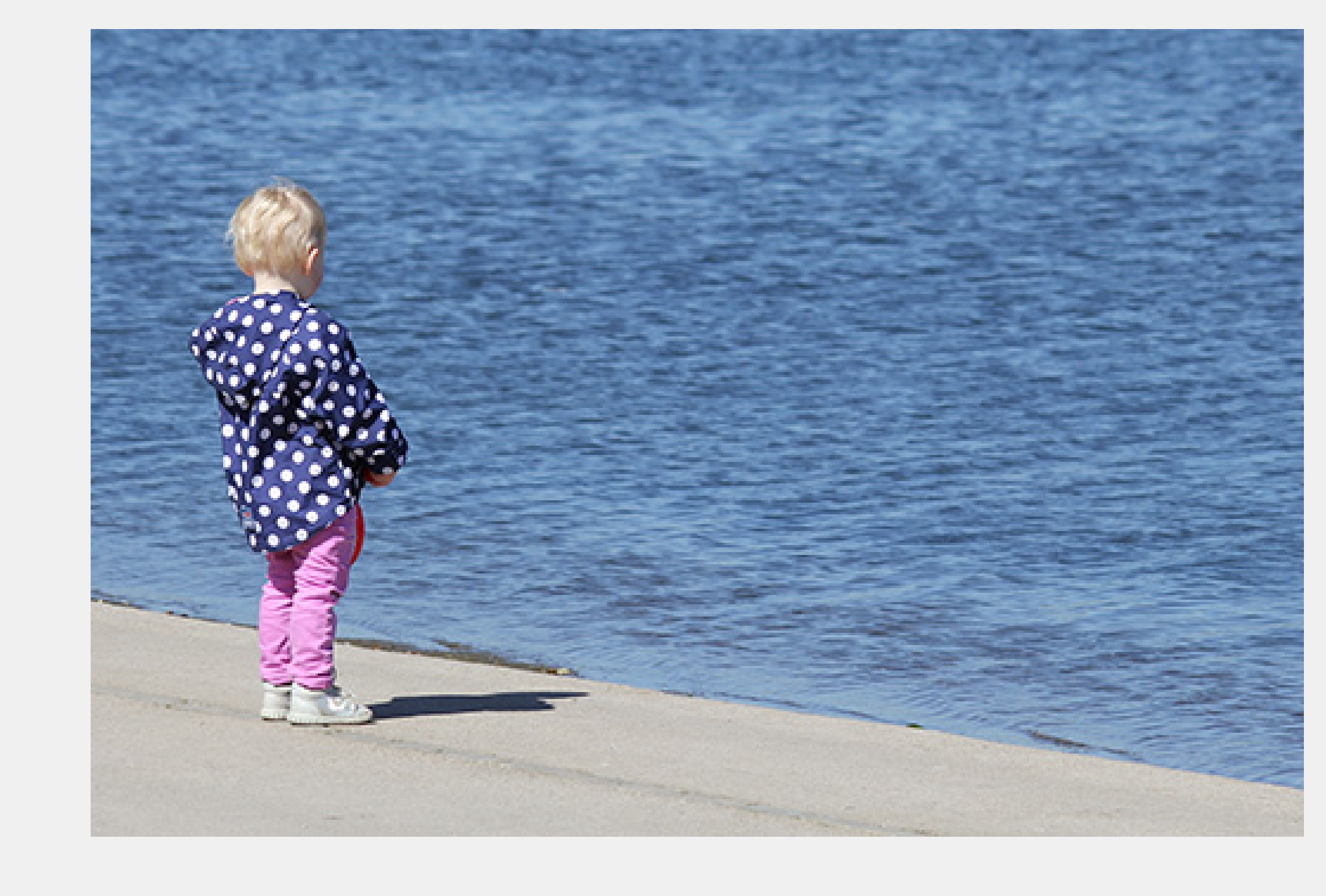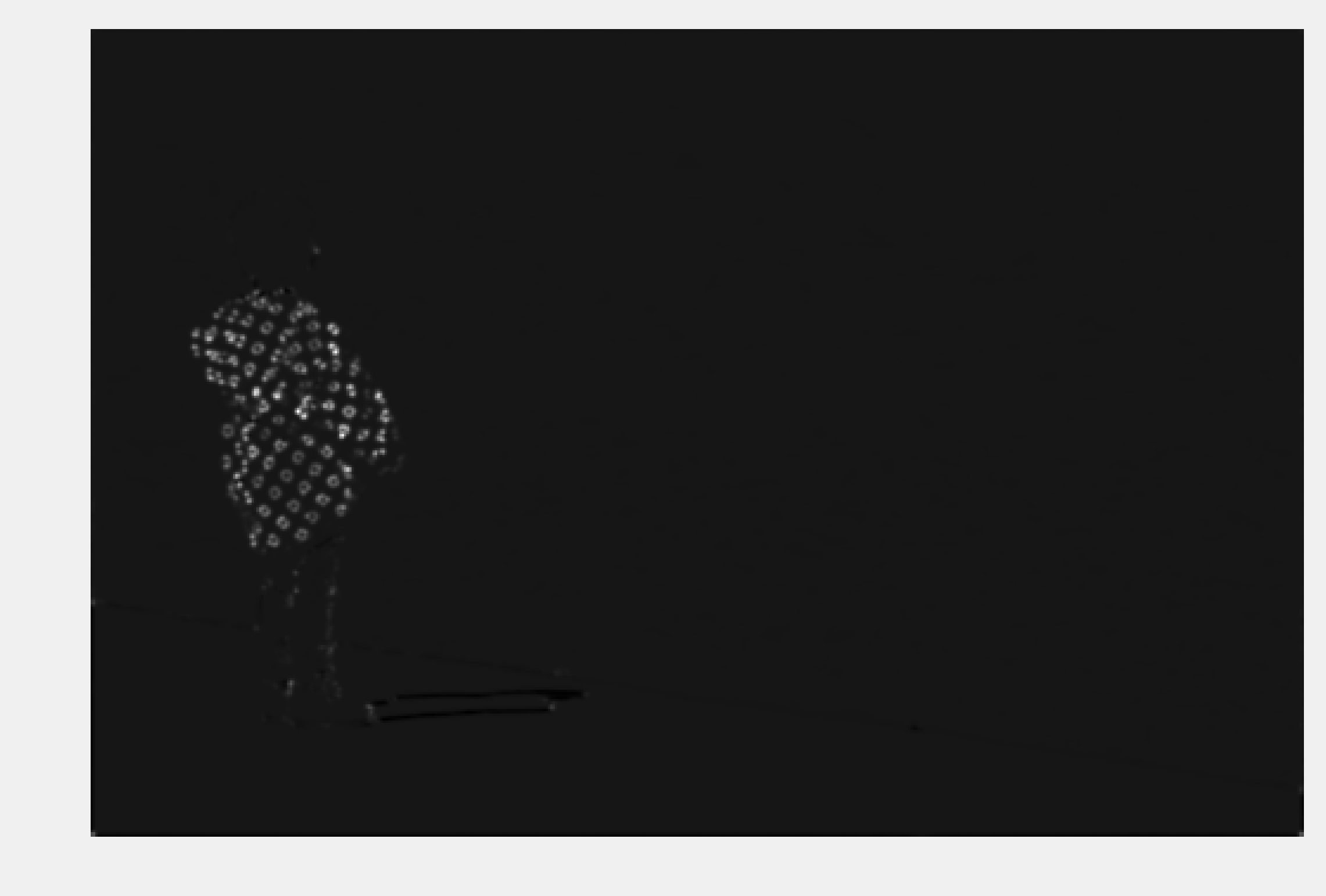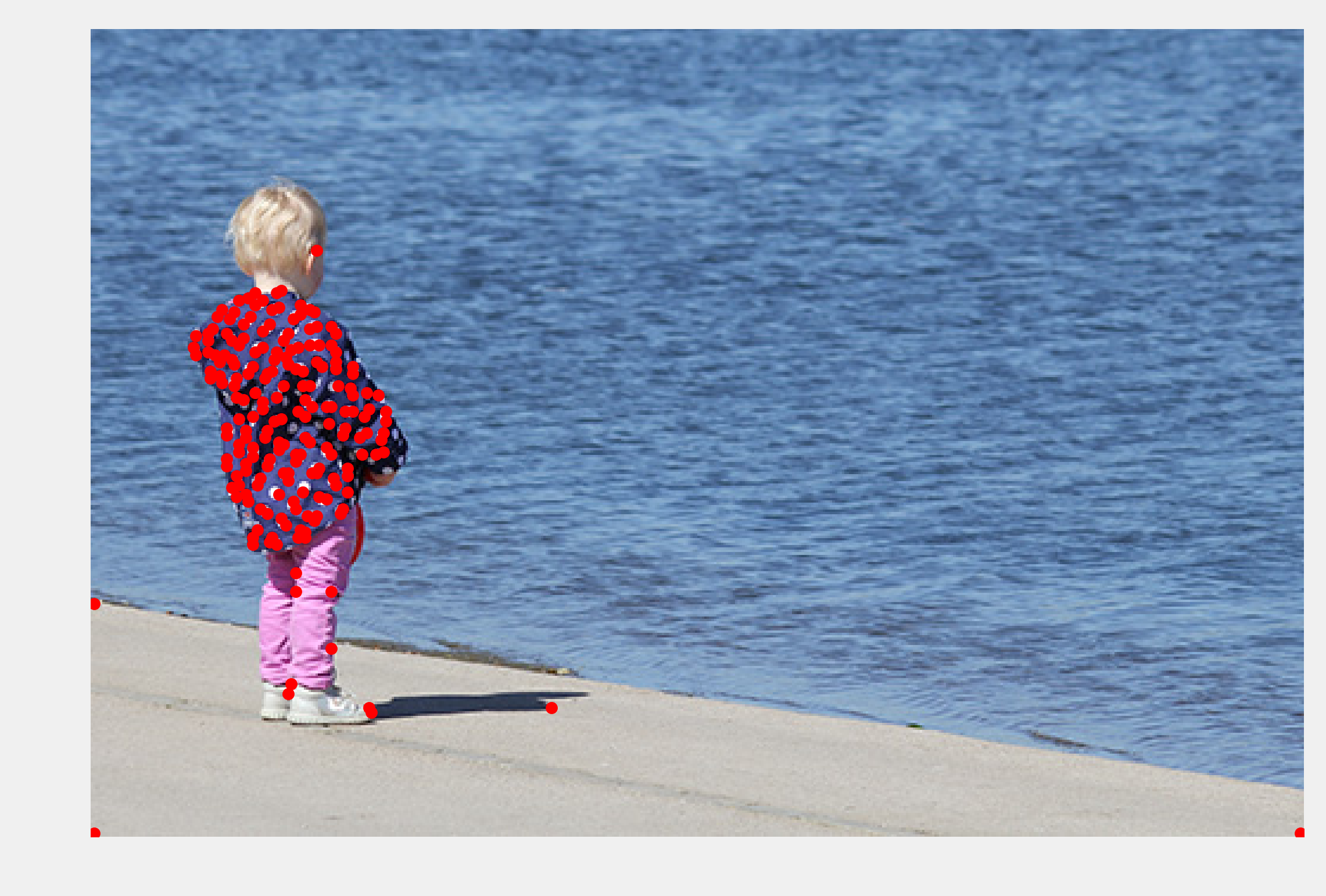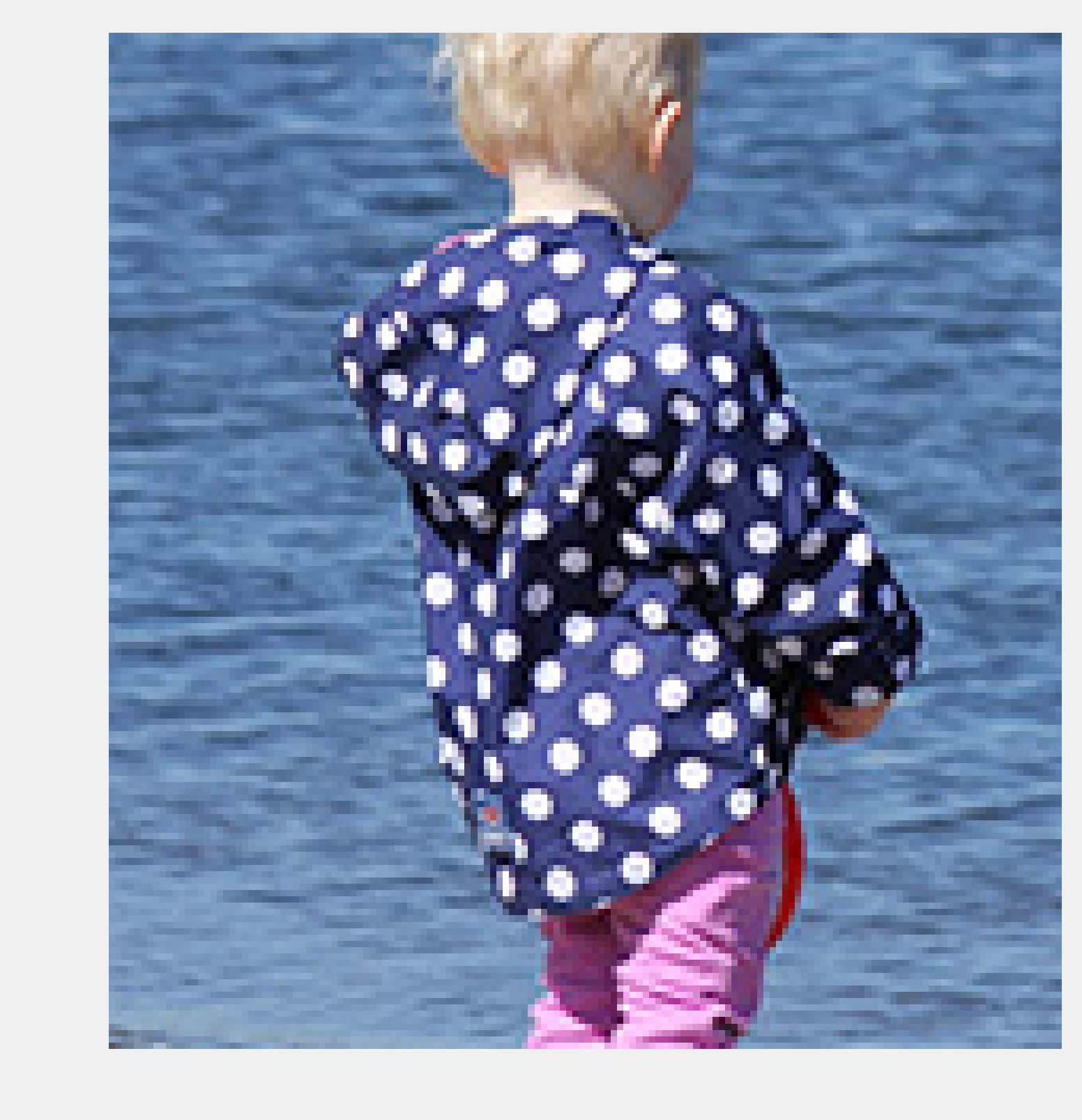This is one of the 100+ free recipes of the IPython Cookbook, Second Edition, by Cyrille Rossant, a guide to numerical computing and data science in the Jupyter Notebook. The ebook and printed book are available for purchase at Packt Publishing.

▶  Text on GitHub with a CC-BY-NC-ND license
▶  Code on GitHub with a MIT license

In an image, points of interest are positions where there might be edges, corners, or interesting objects. For example, in a landscape picture, points of interest can be located near a house or a person. Detecting points of interest is useful in image recognition, computer vision, or medical imaging.

In this recipe, we will find points of interest in an image with scikit-image. This will allow us to crop an image around the subject of the picture, even when this subject is not in the center of the image.

## How to do it...

1.  Let's import the packages:

import numpy as np
import matplotlib.pyplot as plt
import skimage
import skimage.feature as sf
%matplotlib inline


2.  We create a function to display a colored or grayscale image:

def show(img, cmap=None):
cmap = cmap or plt.cm.gray
fig, ax = plt.subplots(1, 1, figsize=(8, 6))
ax.imshow(img, cmap=cmap)
ax.set_axis_off()
return ax


img = plt.imread('https://github.com/ipython-books/'
'cookbook-2nd-data/blob/master/'
'child.png?raw=true')

show(img)4.  Let's find salient points in the image with the Harris corner method. The first step consists of computing the Harris corner measure response image with the corner_harris() function (we will explain this measure in How it works...). This function requires a grayscale image, thus we select the first RGB component:

corners = sf.corner_harris(img[:, :, 0])

show(corners)We see that the patterns in the child's coat are well detected by this algorithm.

5.  The next step is to detect corners from this measure image, using the corner_peaks() function:

peaks = sf.corner_peaks(corners)

ax = show(img)
ax.plot(peaks[:, 1], peaks[:, 0], 'or', ms=4)6.  Finally, we create a box around the median position of the corner points to define our region of interest:

# The median defines the approximate position of
# the corner points.
ym, xm = np.median(peaks, axis=0)
# The standard deviation gives an estimation
# of the spread of the corner points.
ys, xs = 2 * peaks.std(axis=0)
xm, ym = int(xm), int(ym)
xs, ys = int(xs), int(ys)
show(img[ym - ys:ym + ys, xm - xs:xm + xs])## How it works...

Let's explain the method used in this recipe. The first step consists of computing the structure tensor (or Harris matrix) of the image:

$$A = \begin{bmatrix} \langle I_x^2 \rangle & \langle I_x I_y \rangle\\ \langle I_x I_y \rangle & \langle I_y^2 \rangle \end{bmatrix}$$

Here, $$I(x, y)$$ is the image, $$I_x$$ and $$I_y$$ are the partial derivatives, and the brackets denote the local spatial average around neighboring values.

This tensor associates a $$(2,2)$$ positive symmetric matrix at each point. This matrix is used to calculate a sort of autocorrelation of the image at each point.

Let $$\lambda$$ and $$\mu$$ be the two eigenvalues of this matrix (the matrix is diagonalizable because it is real and symmetric). Roughly, a corner is characterized by a large variation of the autocorrelation in all directions, or in large positive eigenvalues $$\lambda$$ and $$\mu$$. The corner measure image is defined as:

$$M = \det(A) - k \times \mathrm{trace}(A)^2 = \lambda\mu - k(\lambda+\mu)^2$$

Here, $$k$$ is a tunable parameter. $$M$$ is large when there is a corner. Finally, corner_peaks() finds corner points by looking at local maxima in the corner measure image.

## There's more...

Here are a few references: